# The Most Beautiful Theorem in Mathematics: Euler’s Identity

## After watching Professor Robin Wilson’s lecture about a Euler’s Identity, I am finally able to understand why Euler’s Identity is the most beautiful equation. To share my fascination with this topic, and reinforce my own knowledge, here are some notes I took during Lewin’s lecture. And you can his beautiful book here.

What could be more mystical than an imaginary number interacting with real numbers to produce nothing? This question was asked by a reader of the magazine Physics World in 2004 to emphasize the beauty of Euler’s equation, “e to the power of i times pi equals negative one”.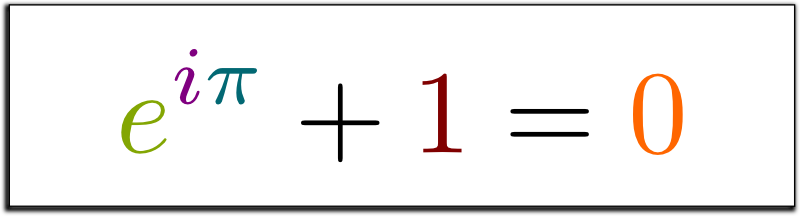Figure 1.0: Euler’s Identity, e to the power of i times pi and then plus one equals to zero.

Before that, in 1988, mathematician David Wells, who was writing for The Mathematical Intelligencer, an American mathematics magazine, listed 24 math theorems and performed a poll asking his readers to choose the most beautiful theorem in his article. And after Euler’s Equation won by a landslide, it has been called “the most beautiful equation in mathematics”.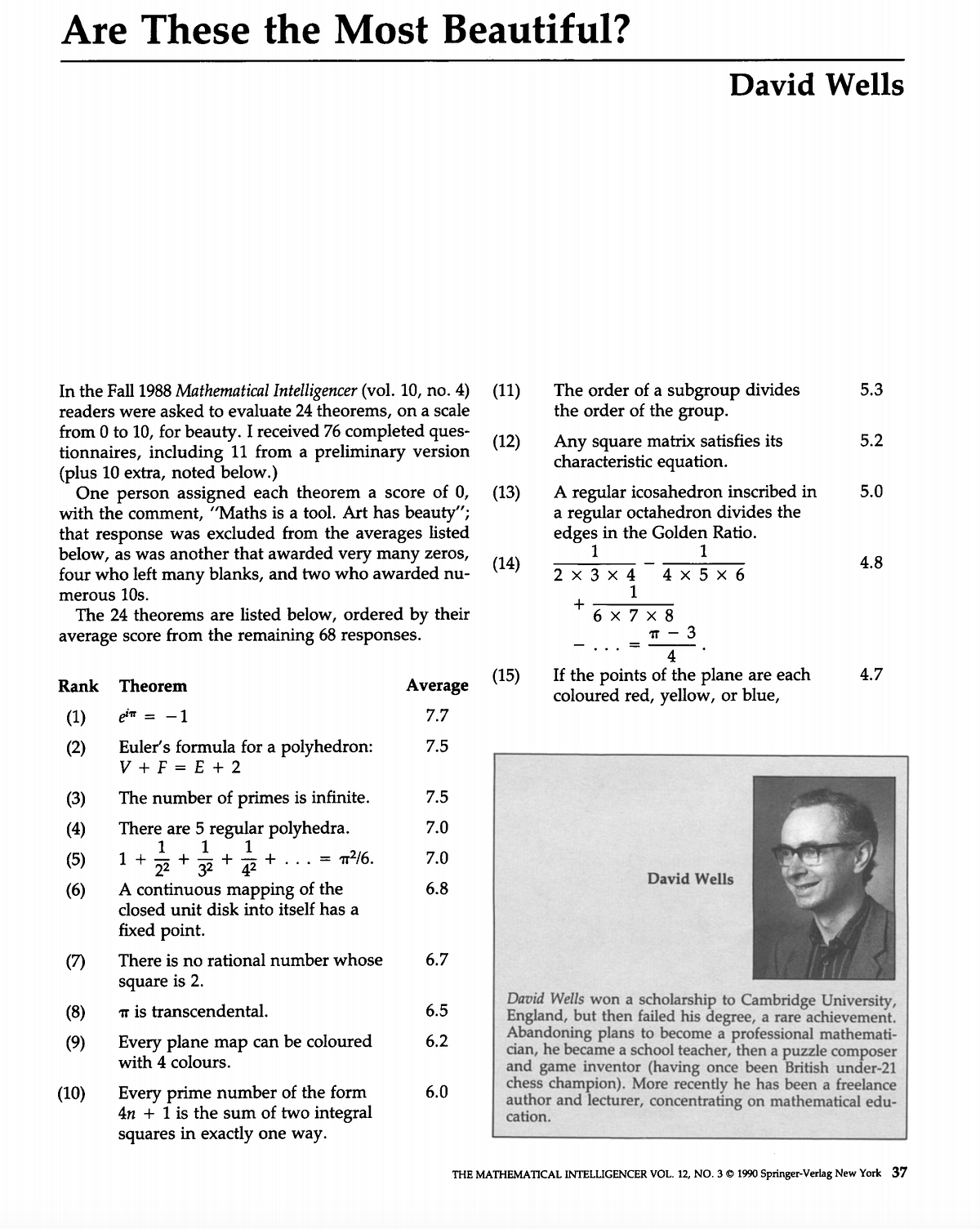Figure 2.0: The cover of The Mathematical Intelligencer magazine, Figure 3.0: David Wells’ poll that appeared in the magazine | | Source: The Springer

Leonhard Euler has been called the most prolific mathematician of all time. Other outstanding mathematicians have been impressed by his works. One of the best physicists in the world, Richard Feynman, described Euler’s equation as “the most remarkable formula in mathematics” in his famous Feynman Lectures on Physics. Another amazing mathematician Michael Atiyah has also described it the formula as “…the mathematical equivalent of Hamlet’s phrase — To be, or not to be — very short, very succinct, but at the same time very deep.”

There are many interesting facts about Euler’s Equation. For instance, it was featured in some episodes of The Simpsons.Figure 4.0: In this scene, the character Lisa is surrounded by books, and Euler’s equation can be seen on the second book on the right-most stack. Figure 5.0: In this scene, a supporting character appears with Euler’s equation on his shirt.

Euler’s equation was also a key point for a criminal court case. In 2003, a Ph.D. candidate at the California Institute of Technology, Billy Cottrell, vandalized sports cars by sprayed Euler’s equation on them. In his trial, his testimonial was: “I’ve known Euler’s theorem since I was five and everyone should know Euler’s theorem.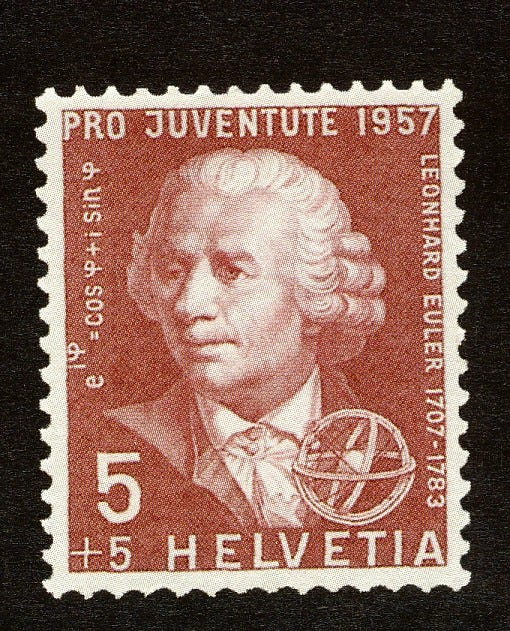Figure 6.0: Bank note issued by Germany in 1983 for the 200th anniversary of Euler’s death (Helvetika), Figure 7.0: A stamp issued by Switzerland in 1957 for the 250th anniversary of Euler’s death (Penn State)

#### Why is Euler’s Equation Important?

A person has a right to ask the question: Why did Billy Cottrell think that everyone should know about Euler’s equation? To the extent that he was willing to spray paint it onto peoples’ cars? The answer is simple. Euler used 3 essential constants in mathematics and applied the mathematical operations of multiplication, then took the powers to write a beautiful formula to get zero or negative 1.

Recall: e to the power of i times pi equals negative one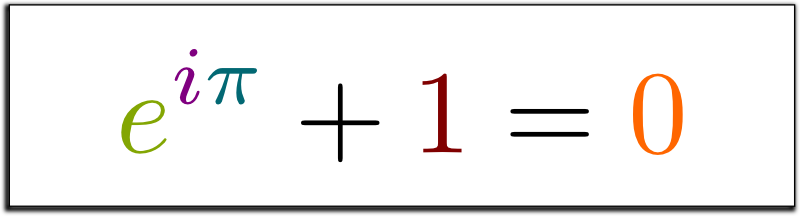Figure 8.0: e to the power of i times pi and plus one equals zero. Image: science4all.org
• The constant number e has a connection with exponential functions.
• The constant number i is not a real number, but an imaginary number which is equal to the square root of negative 1.
• The notorious constant number π (pi) has a connection with circles.

Euler’s identity first appeared in his book Introductio in analysin infinitorum in 1748.. Later, people saw that the formula also had relationships with the trigonometric functions sine and cosine, with surprising results. While the exponential function goes to infinity, trigonometric functions were oscillating between 1 and -1.

e to the power of i times ϕ (phi) = cos ϕ (phi) + sin ϕ (phi)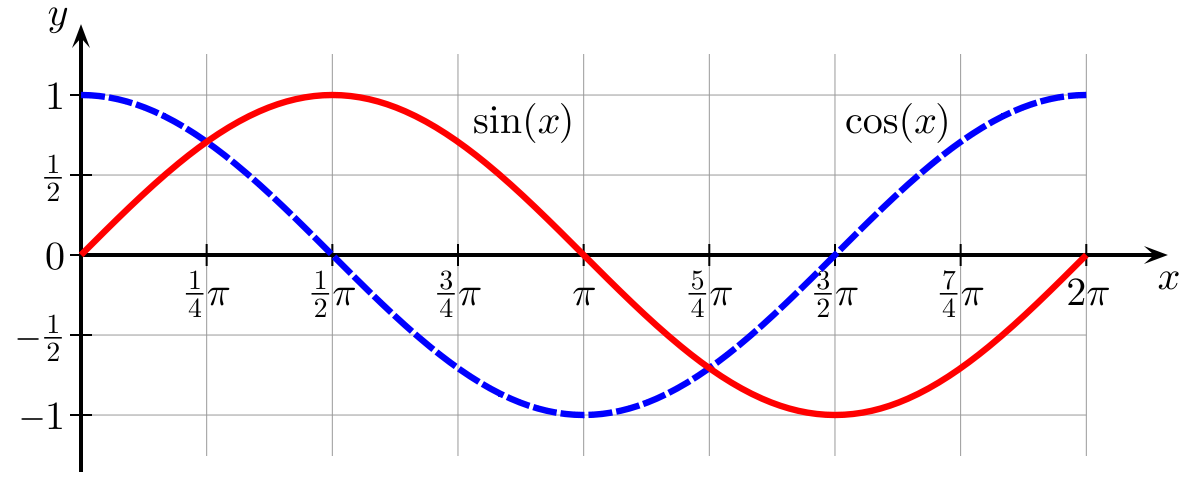Figure 8.0 ( left): The natural exponential function y=ex, Figure 8.1 (middle): the graph of Euler’s Identity, Figure 8.3 (right): The frequencies emitted by an LC circuit.

The equations and graphs displayed above may seem abstract, but they are essential for quantum physics and image processing calculations and are dependent on Euler’s identity.

### 1: The Counting Number

The number one, 1, is the basis of our counting system. We use 1 to start counting. But how do we count? To count, we use the digits from 0–9 and a place value system which determines the value of a digit.

For instance, the number 323 means 3 hundred, 2 tens and 3 ones. Here, number 3 plays two different roles depending on its position.

23 = (3100) + (210) + (31)

There is also another counting system which is called the binary system. This system uses base 2 instead of base 1. This is commonly used by computers and in programming. For example, in a binary system:

1001 = (1²³) + (0²²) + (0²¹) + (1²⁰) = [9 in the base-1 system]

Who created the counting system? How did the first humans count their objects or animals?

How did our counting systems arise? How did early civilizations count? We are confident that they were not using our place value system from the beginning. For instance, 4000 years ago, Egyptians were using a counting system with different symbols. However, they were combining the symbols to create a new symbol to represent the numbers.Figure 11: The hieroglyphs here form the number 4622, one of the numbers carved on the wall of the temple in Karnak, Egypt. Source: Futurelearn, Figure 12: Hieroglyphs were pictures used to represent words, or, in this case, numbers.

At the same time, at a different place, another society also found a solution for counting, but again, they were also using symbols. Additionally, their counting system was base 60, not 10. We use their counting method for determining time; hence the basis for 60 seconds in a minute and 60 minutes in an hour.Figure 13: Babylonian numerals, based on the sexagesimal (base-60) number system. Source: Wikipedia

A thousand years later, Romans invented Roman numerals. They used letters to represent numbers. Roman numerals are not considered a place system because they used different letters for a lot of value in our counting system. This is also the reason why they used an abacus for counting.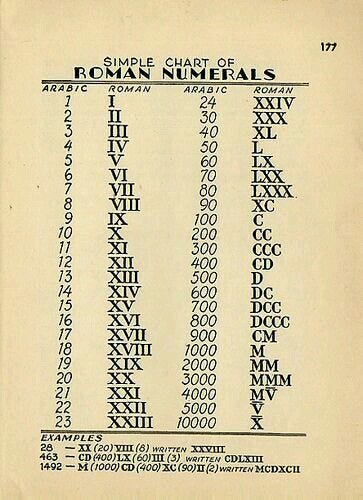Figure 14: A Romanesque Abacus, using a hexadecimal (base-16) notation. Source: DK Learning, Figure 15: Chart of conversion from Arabic to Roman Numerals

Ancient Greek numbers did not use a place value system either. Greek mathematicians used letters for numbers. They had special letters for numbers from 100 to 900. Most of the people at the time found Greek numbers confusing.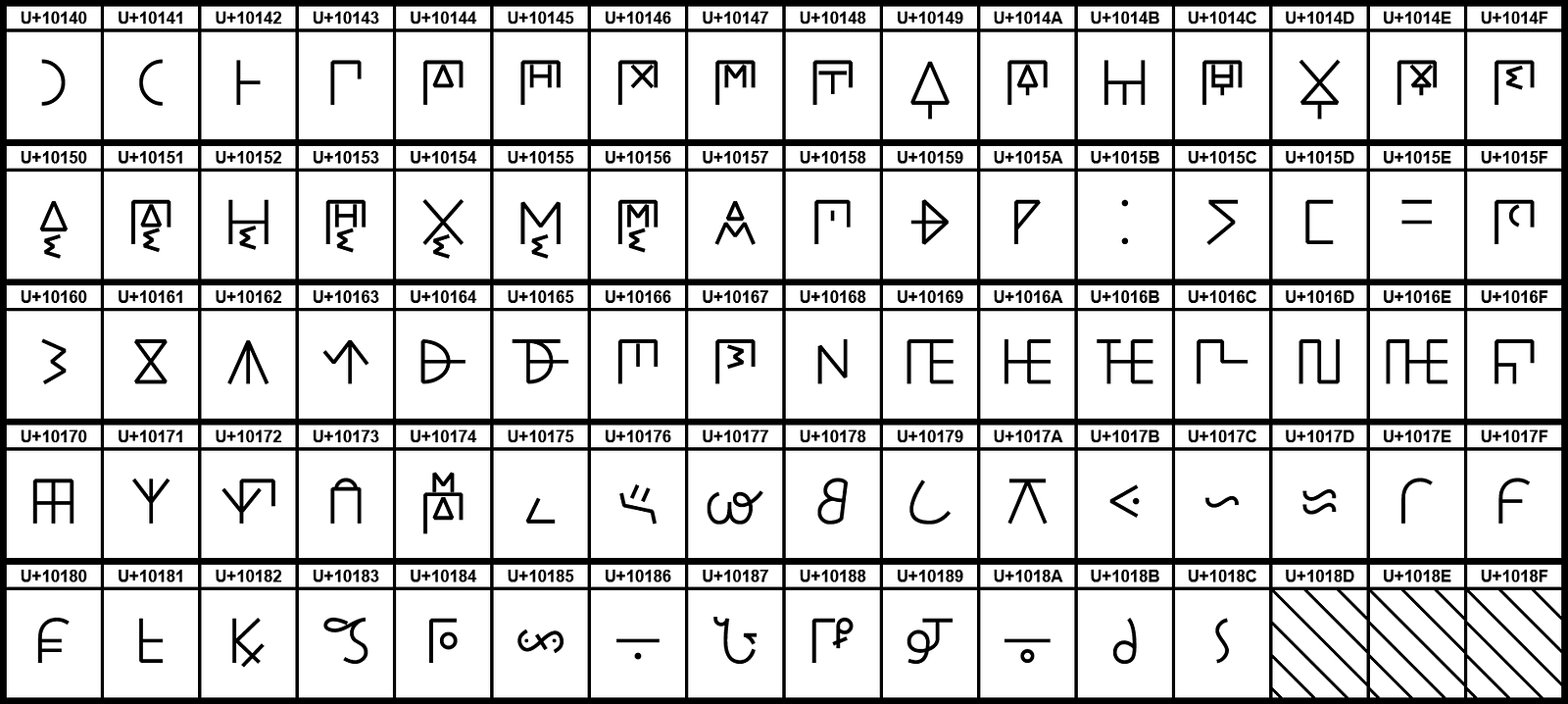Figure 15: A chart of Ancient Greek letters. Source: Wikimedia

At the same time, Chinese mathematicians started using small bamboo rods for their counting operations. This Chinese counting method is accepted as the first decimal place value system.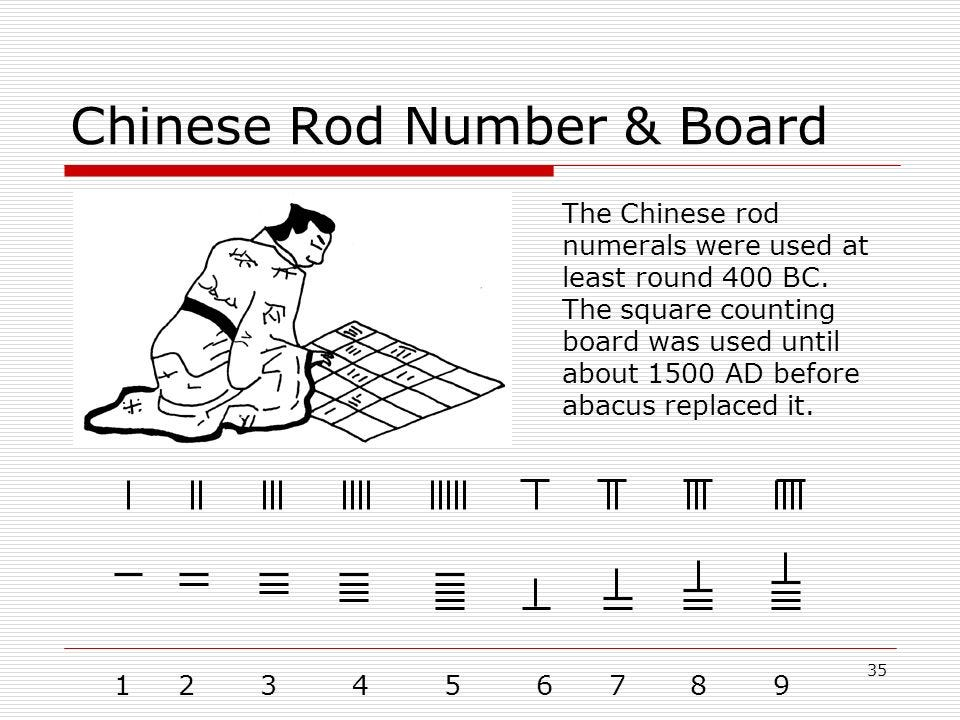Figure 16: The Chinese counting method that used rod numerals.

However, the most unique method was used by The Mayans. Their counting system was base-20. They used dots and lines to represent numbers from 1 to 19. What made their counting system different? They used a pictorial head for each number, and a symbol for zero, 0.Figure 17: The Mayan base-20 counting system, using heads to represent different numbers. Figure 18: An alternative way of writing Mayan numerals. Source: Wikipedia

### 0: A number from nothing

Some civilizations used spaces to distinguish a number like 101 from 11. After a while, a special number, zero, began to emerge. For instance, in the cave in Gwalior in India, archaeologists discovered the number 270 was on the wall, and they also saw the number zero. The earliest recorded use of zero can be seen in the Bodleian Library.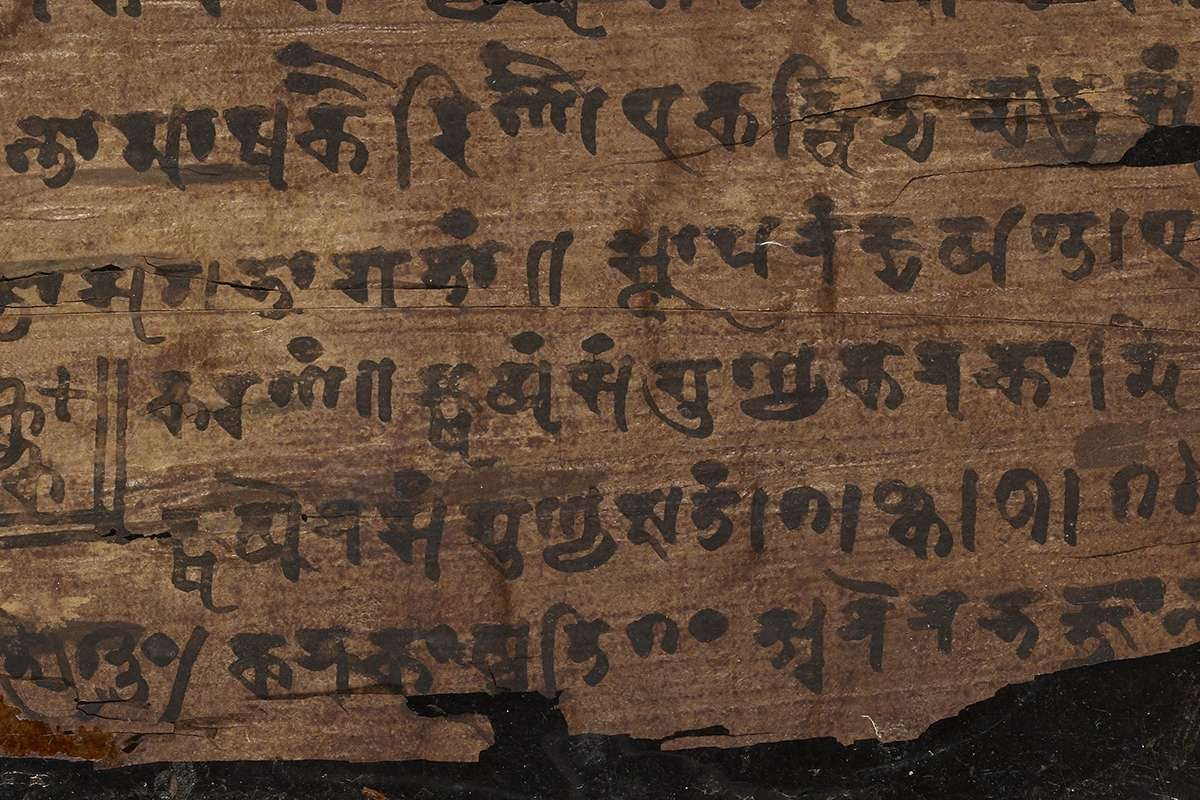Figure 19: Found carved into the wall of a temple in Gwalior, India, the circle in the middle represents ‘zero’. Approximately 1500 years old. Source: The Guardian Figure 20: The black dots on the Bakhshali manuscript represent ‘zero’, and are the oldest recorded usage of the number at approximately 1800 years old. Source: BBC

Almost 1400 years ago, the rules for calculating with zero were laid out. For instance, if you added a negative number and zero, you would get a negative number. Division by zero was not allowed because if you can divide by zero, you would get a number could be equal to any number you wanted, which should not be possible.

Soon afterward, arithmetic books promoting Hindu-Arabic numerals were published by many people. You can see the evolution of Hindu-Arabic numerals below. Most of the countries used the Hindu-Arabic numeral system, but Arab countries still used Arabic numerals.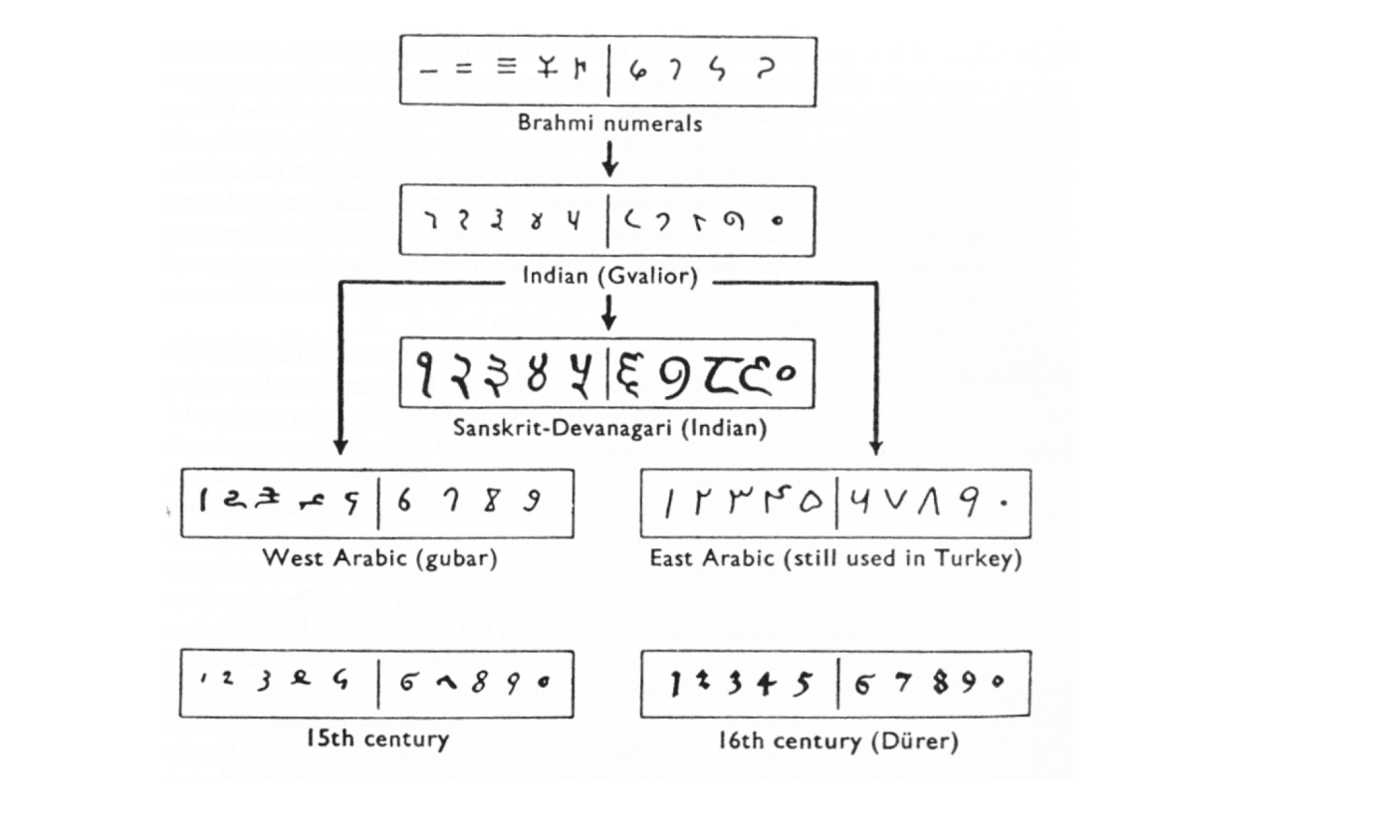Figure 21: This chart depicts the evolution of numerals, stemming from Brahmi numerals and ending with the numerals still used today. Source: Languages as Symbols Figure 22: The classic engraving of Arithmetica from Gregor Reisch’s Margarita Philosophica, depicting the contest between Boethius [left], smiling after discovering Hindu-Arabic numerals and written calculation, and Pythagoras [right], frowning, still using a counting board. | Source: Bit by Bit

#### Pi (π): The notorious irrational number

Pi is the most popular irrational known today. We can find pi in two different ways; the ratio of the circumference of a circle to its diameter, or the ratio of the area of a circle to the square of its radius. Euclid proved that these ratios are always constant for all circles, even the moon, a penny, a tire, etc.

#### π = circumference / diameter OR π = area of a circle / radius²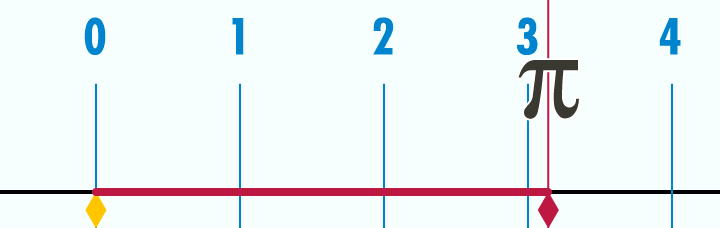Figure 22: The relationship between circumference and diameter in relation to pi, visualized in a gif. Source: Reddit

Since irrational numbers, like pi, are non-terminating, and without a pattern, we can never finish writing pi. It goes on forever. There are some dedicated people who memorized many decimal places (with the current world record being at 70,000 digits! Source: Guinness World Records ).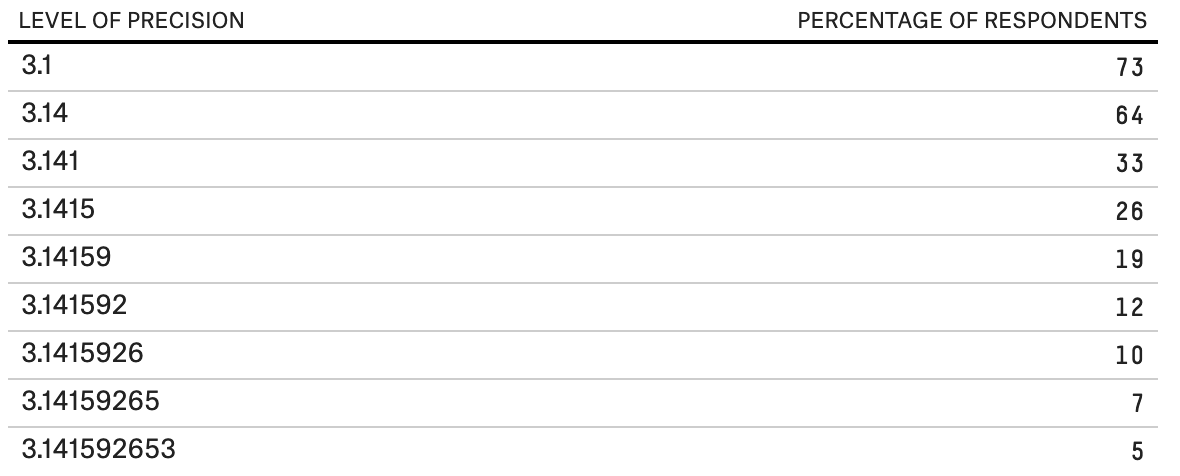Figure 23: A poll performed 941 respondents to determine the percentage of people that can say pi after the decimal point. Source: FiveThirtyEight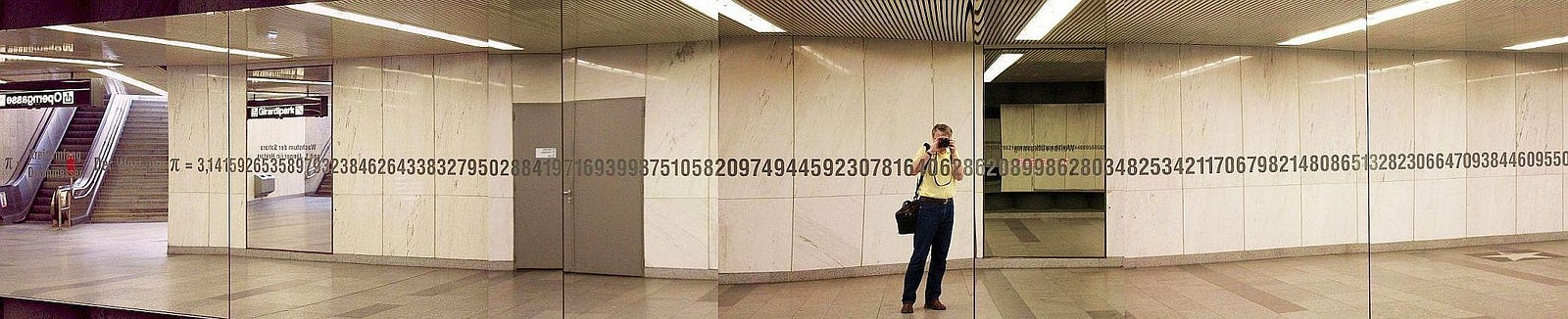Figure 24: At the Karlsplatz metro station, Vienna, hundreds of digits of pi are inscribed on the wall. Source: Akropolis

So far, computers have only calculated 2.7 trillion digits of pi (Source: BBC). It may seem like a lot, but there’s still a long way to go.

Earlier, it was mentioned that Euclid found the number pi. What were people doing before Euclid when they needed to find the area of a circle? Historians found a clay tablet from Babylon, which related the ratio of the perimeter of a hexagon to the circumference of the surrounding circle. After some calculations, the number found was 3.125. Very close to pi.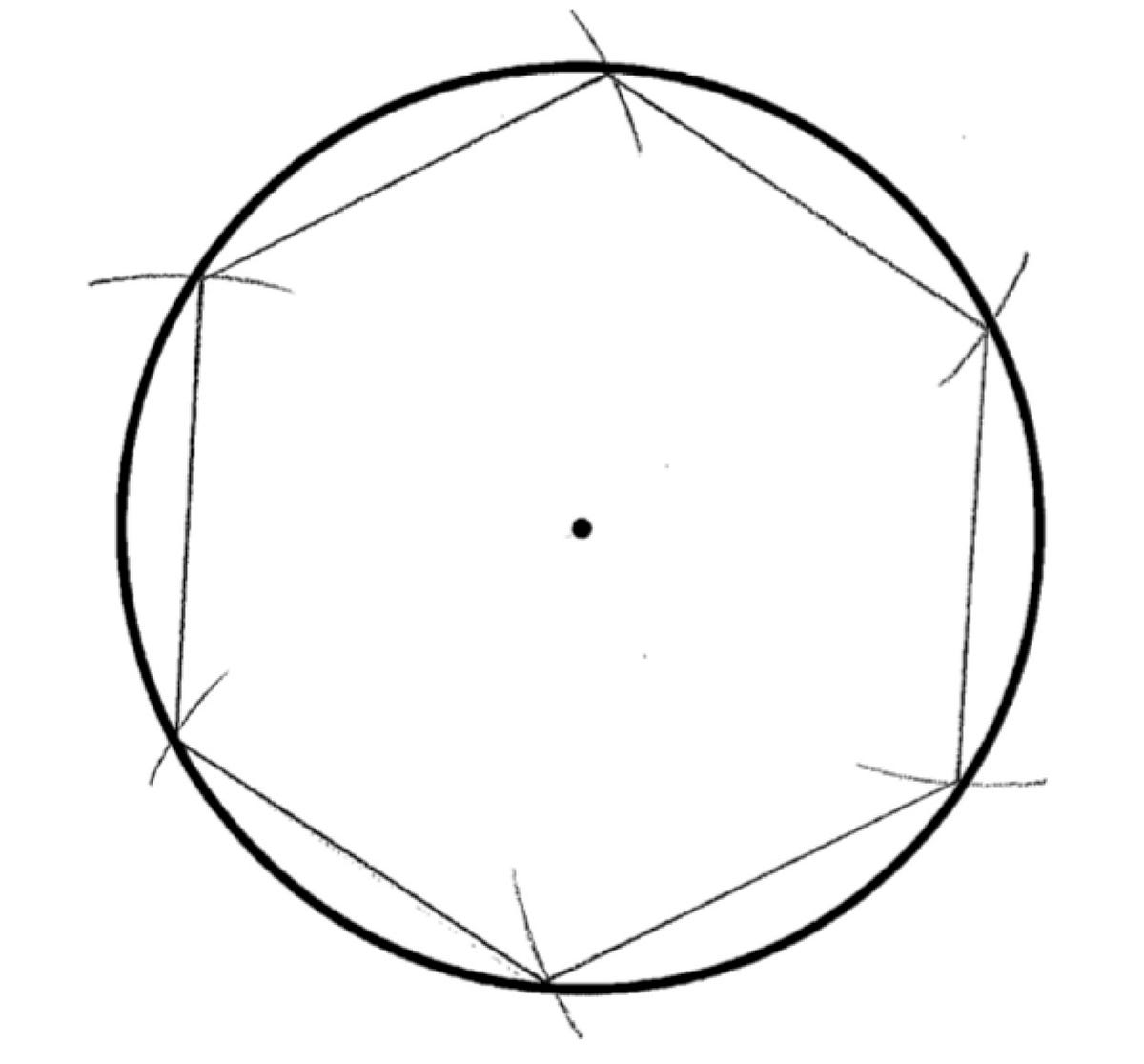Figure 24: clay tablet from Babylon, which related the ratio of the perimeter of a hexagon to the circumference of the surrounding circle. Figure 25: Numberwarrior

The Egyptians also got very close to pi. Historians found a document which shows us how Ancient Egyptians found the ratio of pi. When historians translated the document they saw this question:

For Instance, to find the area of around the field with a diameter of 9 khet (1 khet = 57.2 yards), the area is calculated as follows:-
Take away 1/9th of the diameter, namely 1. The remainder is 8. Multiply it by 8 which gives 64. Therefore, the area will be 64 setjat (unit of area).
In other words, the diameter is equal to 2r, and 1/9 of the radius is (1/9 • 2r), then if we subtract it from the original diameter, we get:
2r — (1/9 • 2r) = 8/9(2r). Then the area of the circle will be 256/81 r². So pi is almost 3.16. They discovered this value of pi almost 4000 years ago.

However, some Greek mathematicians found a better way to find pi. For instance, Archimedes preferred to work with perimeters. He started drawing circles outside the different size of polygons. When he had a hexagon, he drew a circle with the diameter 1. Then he saw that each side of the hexagon is 1/2 and the perimeter of the hexagon 1/2 x 6 = 3. Then, he was increasing the number of the side of polygons until it became almost a circle. When he worked with a 96-sided polygon and applied the same method, he got 2 decimal places of pi, 3 and 10/71 = 3.14084. After many years, Chinese mathematician Liu Hui used 3072-side polygon and got a number 3.14159, 4 decimal of pi. After that, another Chinese mathematician Zu Chongzhi did more impressing work. He worked with 24,000-side polygon and he got 3.1415926, six decimal places of pi.

After a thousand years, a German mathematician Ludolph van Ceulen worked with ²⁶²-side polygon and he got 35 decimal places of pi. And his number, Ludolphian number, still appears on his tombstone.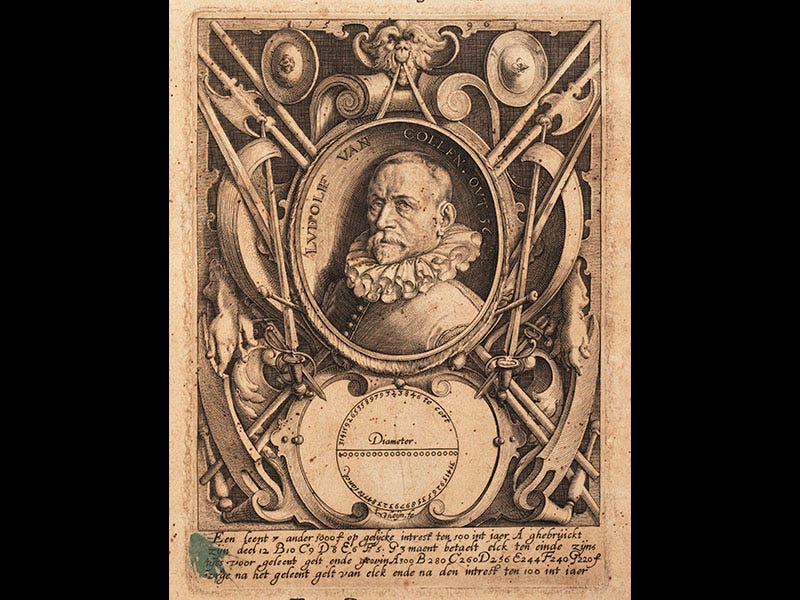Figure 27: Claymath | Figure 28: Lindahall

Indeed in 1861a gentleman from Potsdam use these very series to find pi to 261 decimal places. Other times the minus 1 results where the series converge even faster.

In 1706, the Englishman John Machin, professor of astronomy for many years, used the addition formula to prove that pi is

Without worrying about how he got it, he just used that formula over and over again and then he wrote out the series shown here. It was the greatest improvement for pi so far.Figure 29: Machin’s formula for pi

However, the first time that pi ever appeared was in 1706. A math teacher, William Jones, wrote a book and he introduced pi for measuring circles for the first time. It was the first time pi appeared on a book!

In 1873, William Shanks used John Machin’s formula and obtained 707 decimal places of pi. You can see those numbers inscribed in the pi room of the Le Palais de la découverte. However, then mathematicians realized that only 527 decimals places were correct.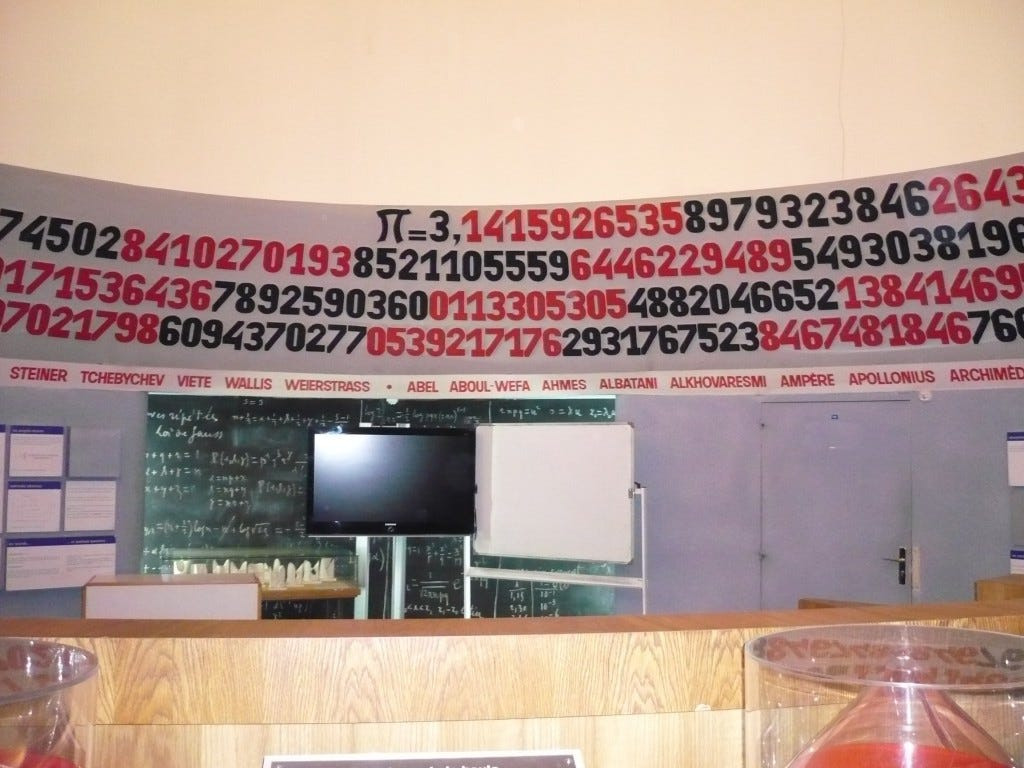Figure 31: Source: Les maths autrement

On the other hand, Buffon found the most interesting method to find pi. His experiment based on throwing toothpicks randomly to estimate pi. He drew some parallel lines on a board with a distance D apart and used needles with the length of L. When he threw the needles randomly on the board, he recorded the proportion of needles which the cross a line.

And after that, another mathematician Lazzarini tossed a needle 3408 times and he got six decimal places of pi, π with the ratio 355/113. However, if one needle didn’t cross a line he would only get 2 decimal places of pi.Figure 32: Throwing 1000 needles to estimate pi | Source

#### e: The History of Exponential Growth

e is the other famous irrational number. The decimal part of e also goes forever like pi. We use the number e for exponential growths. In other words, we use e if we see something grows or decreases very fast.

One of the greatest, maybe the best, mathematician Leonard Euler, discovered the number e in 1736 and he mentioned his special number in his book, Mechanica, for the first time.

To understand exponential growth, we can use the story about the inventor of chess. When the chess guy finished his game, he showed it to the king of the North. He was not Jon Snow at this time. Anyway, the king loved the game and promised that he would give the chess guy whatever he wants as a reward. So the chess guy demanded something very simple; ¹² grains for the first square of the chessboard, ²² grains for the second square of the chessboard, ³² grains for the third square of the chessboard, and so on. The amount of the grain was doubling each time. The king in the north thought that it was easy for him but he was wrong because, for the last square part, he needed to give ²⁶³ which were equal to 9,223,372,036,854,775,808 grains. That was exponential growth. It started with 1, kept doubling, and after 64 steps, it became something huge!!!

If the chess guy chose a linear equation such as 2n, then he would get 2, 4, 6, 8, …, and 128… Thus, in the long run, exponential growth tends to exceed polynomial growth often by a huge margin.

By the way 9,223,372,036,854,775,808–1 is the is the maximum value for a 64-bit signed integer in computing.

Euler invented the number e. However, Jacob Bernoulli was also dealing with the number e while he was calculating continuous compound interest to make more money. So, if we invest our \$100 at a 10% interest how does it grow? First of all, it depends on how often the bank calculates the interest. For instance, if they calculate one time, then we get \$110 at the end of the year. If we change our mind and decide to take our money every 6 months, this time we will get more than \$110. The reason for that, this time the interest you get from the first 6 months will also get another interest. Interest is getting interested. Interesting! Our total money will be \$110.25. If we approach intuitively, we can see that we can get more money if we take our money every quarter of a year. And if we make the time frame shorter and shorter, final amounts are increasing. Thus continuous compound interest makes us rich! However, our total interest is approaching a limiting value which is related to e.

Bernoulli didn’t call the number 2.71828 as e. When Euler worked on 2.71828, he got the exponential function e to the power of x. He put his findings in his book, The Analysis of Infinite.

In 1798, Thomas Malthus used the exponential function for in his essay which was about food shortages in the future. He made a linear graph to show the increase of production food and made an exponential growth to show the population of the world. In his conclusion, he noted that exponential growth would win in the long term and there would be severe food shortages in the world. And this is what is called the Malthusian catastrophe. Newton was also used the same model to show cooling of his cup of tea.Figure 35: Newton’s Law of Cooling, Figure 36: Malthusian Catastrophe

### Imagining a Number: i, the Square Root of -1

Mathematicians had solved their problems with just using ordinary numbers for a long time. However, after one point, to step further, they needed to discover something new and mysterious. For instance, the Italian mathematician Cardano was trying to separate the number 10 into 2 parts whose product is 40. To solve this, he wrote an equation: x (10-x) = 40. When he solved this quadratic equation, he got the solution to be 5 plus the √-15 and 5 minus √-15 which was meaningless at that time. It was meaningless because, by the definition of square root, he needed to find a number which is square was negative. However, positive times positive and negative times negative are both positive. Anyway, he found his unique number. However, Euler was the first mathematician named √-1 (square root of negative one) as i, the imaginary number.

Leibniz made a comment about the imaginary number √-1:

The imaginary numbers are a wonderful flight of God’s Spirit: they are almost an amphibian between being and not being.

We can add, subtract, multiply, and divide imaginary numbers. Addition, subtraction, and multiplication are straightforward, but the division is a little bit complicated. We add real numbers and imaginary numbers separately. For multiplication, i² is going to be -1.

After Euler, another mathematician Caspar Vessel represented imaginary numbers geometrically and made the complex plane. Today, we represent each complex number a + bi as a point with coordinates (a,b).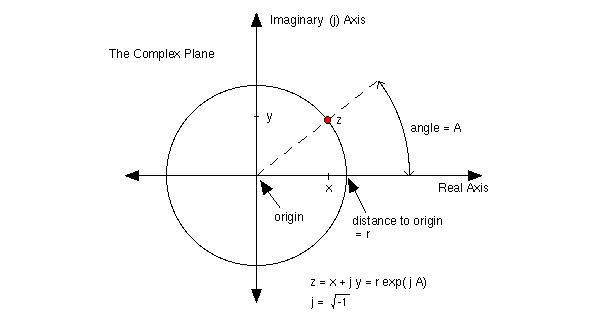Figure 37 &38: The Complex Planes Source

There was a lot of suspicion in Victorian times about these imaginary numbers. However, the Irish mathematician and astronomer, William Rowan Hamilton ended the suspicion by defining the complex numbers in terms of quaternions.

### The Most Beautiful Equation: Euler’s Identity

Euler’s identity connects the exponential function where the functions cosine and sine which oscillate between 1 and -1. To find the connection of trigonometric functions and we can expand them as infinite series which are valid for all values of